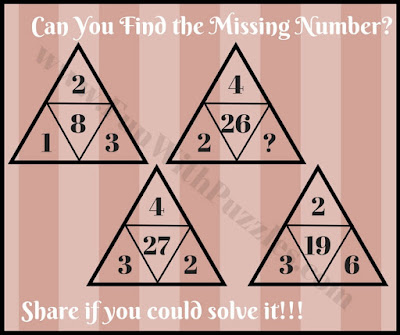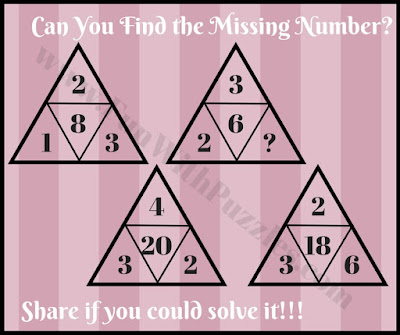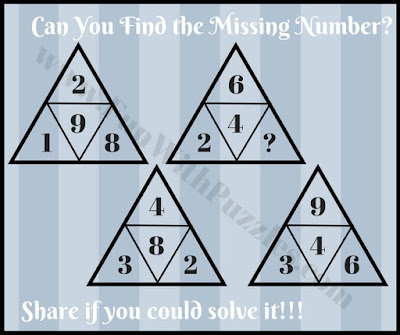Earlier we have published Triangles Math Puzzles in which one has to find the missing number which will replace the question mark. This post is an extension of that Triangle Math Puzzles. Here are the puzzles which are mind-twisting, tricky, tough, and brainbuster as compared to previous puzzles. If you are looking for similar Math Brain Teasers you should check Star Maths Puzzles, and Circle Math Puzzles.
In these Brain Buster Math Questions, you are given four triangles. Each triangle contains four numbers that relate to each other with some arithmetic operation. Your challenge to find this arithmetic operation between these numbers and then find the missing number in one of the triangles using the same arithmetic operation.
Answers to these brain buster maths questions are given at the end. Do post your answers with an explanation in the comment section.1. Simple free math triangle puzzle question2. Brain busting free math triangle puzzle question3. Brain twisting free math triangle puzzle question4. Tough free math triangle puzzle question5. Tricky free math triangle puzzle question